Question

# 4. . The reaction 2NO(g) + O2(g) → 2NO2(g) has the following rate law: Rate =...

4. . The reaction 2NO(g) + O2(g) → 2NO2(g) has the following rate law: Rate = k[O2][NO]2 .

If the concentration of NO is reduced by a factor of two, the rate will __________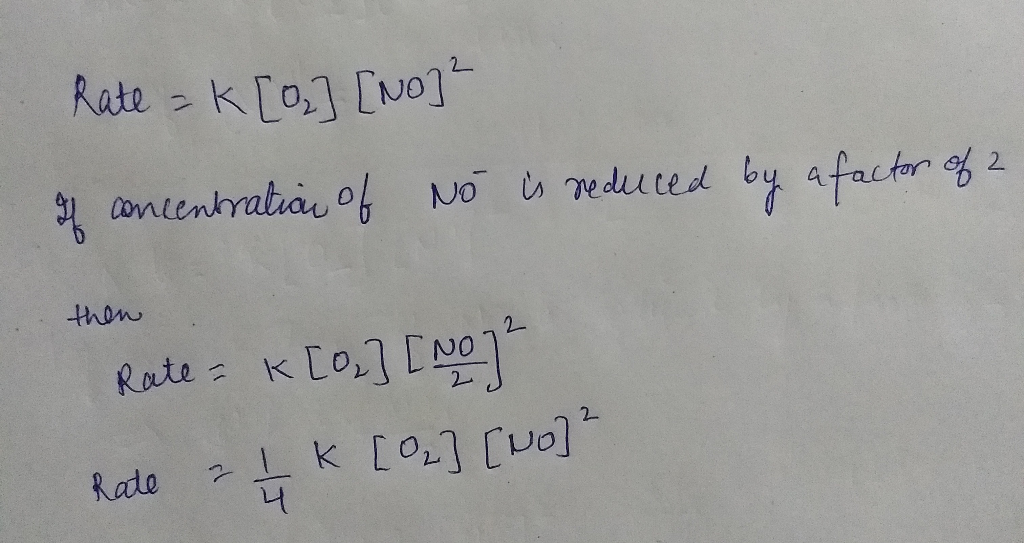#### Earn Coins

Coins can be redeemed for fabulous gifts.

Similar Homework Help Questions
• ### 3. Consider the following reaction and its rate law. Answer the following questions. 2NO(g) + O2(g)...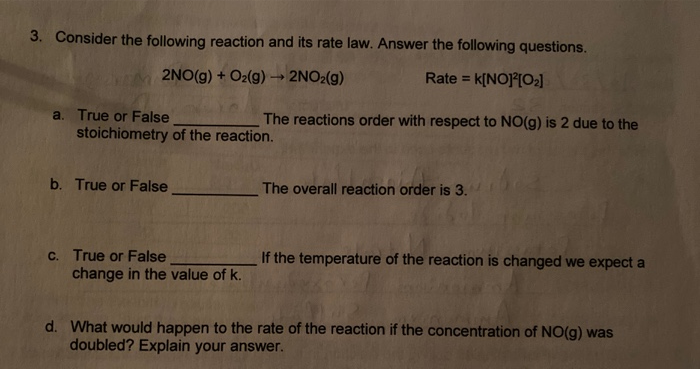3. Consider the following reaction and its rate law. Answer the following questions. 2NO(g) + O2(g) → 2NO2(g) Rate = k[NO]  a. True or False The reactions order with respect to NO(g) is 2 due to the stoichiometry of the reaction. b. True or False The overall reaction order is 3. C. True or False change in the value of k. If the temperature of the reaction is changed we expect a d. What would happen to the rate...

• ### Consider the following reaction: 2NO(g)+O2(g)⇌2NO2(g)2NO(g)+O2(g)⇌2NO2(g) The data in the table show the equilibrium constant for this...

Consider the following reaction: 2NO(g)+O2(g)⇌2NO2(g)2NO(g)+O2(g)⇌2NO2(g) The data in the table show the equilibrium constant for this reaction measured at several different temperatures. Temperature K 400 1.9x10^7 425 2.5x10^6 465 1.6x10^5 515 8.8x10^3 600 2.0x10^2 Use the data to find ΔrS∘ΔrS∘ for the reaction. (answer in J K-1 mol-1) The answer is NOT 158

• ### Consider the following reaction: 2NO(g)+O2(g)→2NO2(g)2NO(g)+O2(g)→2NO2(g) Estimate ΔG∘for this reaction at each of the following temperatures and...

Consider the following reaction: 2NO(g)+O2(g)→2NO2(g)2NO(g)+O2(g)→2NO2(g) Estimate ΔG∘for this reaction at each of the following temperatures and predict whether or not the reaction will be spontaneous. (Assume that ΔH∘ΔH∘ and ΔS∘ΔS∘ do not change too much within the give temperature range.) 1) 713 2) 852

• ### 2. (10 pts) Consider the following second-order reaction: 2NO2(g) + 2NO(g)+O2(g) The rate constant is 0.54...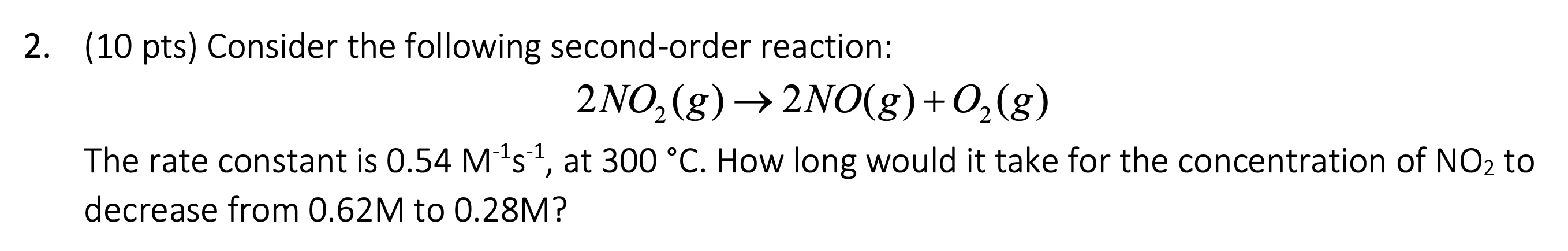2. (10 pts) Consider the following second-order reaction: 2NO2(g) + 2NO(g)+O2(g) The rate constant is 0.54 M-15-1, at 300 °C. How long would it take for the concentration of NO2 to decrease from 0.62M to 0.28M?

• ### The equilibrium constant KcKc for the reaction 2NO(g)+O2(g)⇌2NO2(g)2NO(g)+O2(g)⇌2NO2(g) is 6.9×1056.9×105 at 500 KK. A 5.0 LL...

The equilibrium constant KcKc for the reaction 2NO(g)+O2(g)⇌2NO2(g)2NO(g)+O2(g)⇌2NO2(g) is 6.9×1056.9×105 at 500 KK. A 5.0 LL reaction vessel at 500 KK was filled with 0.052 molmol of NONO, 1.1 molmol of O2O2, and 0.90 molmol of NO2NO2. Part A What is the value of QcQc? Express your answer to two significant figures.

• ### Calculate the enthalpy of the reaction 2NO(g) + O2(g)2NO2(g) Hess's law states that "the heat released or absorbed in a...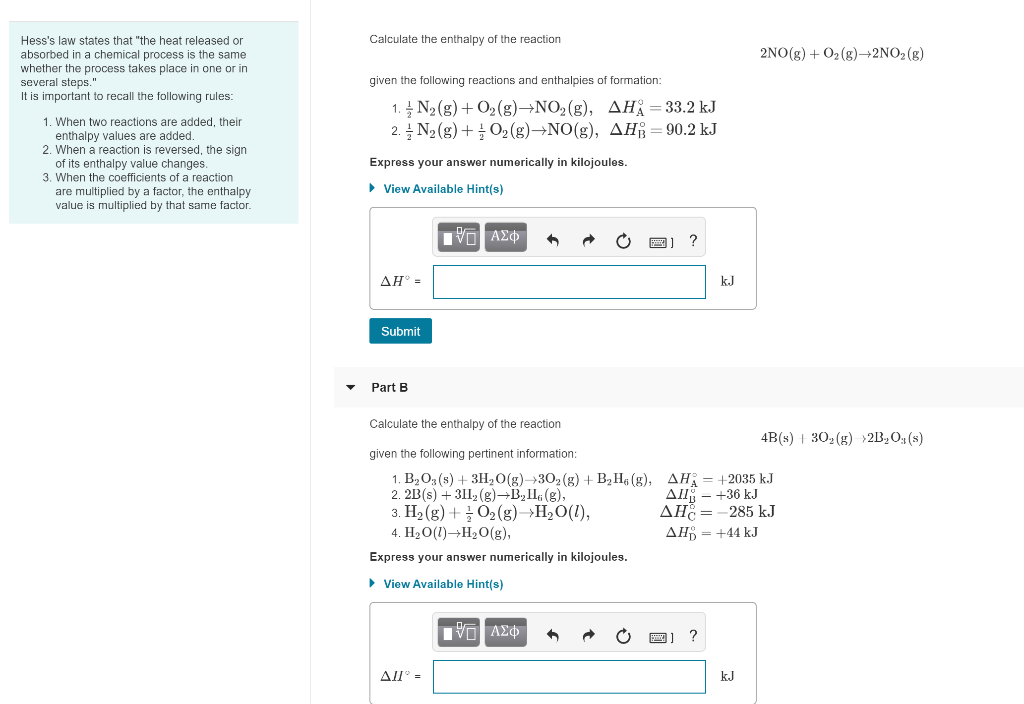Calculate the enthalpy of the reaction 2NO(g) + O2(g)2NO2(g) Hess's law states that "the heat released or absorbed in a chemical process is the same whether the process takes place in one or in several steps." It is important to recall the following rules: given the following reactions and enthalpies of formation: 1. ¢ N2(g) + O2(g)+NO2(g), AH; = 33.2 kJ 2. N2(g) + O2(g) NO(g), AHB = 90.2 kJ 1. When two reactions are added, their enthalpy values are...

• ### Using The Following Data, Determine the Rate Law For The Reaction. Part A. 2NO(g)+O(g) --> 2NO2(g)...

Using The Following Data, Determine the Rate Law For The Reaction. Part A. 2NO(g)+O(g) --> 2NO2(g) Experiment [NO] [O2] Initial Rate (M/s] 1 0.0126 0.0125 1.41x10^-2 2 0.0252 0.0250 1.13x10^-1 3 0.0252 0.0125 5.64x10^-2 Part B. S2O8charge2-(ag)+3 I charge 1-(aq) ----> 2SO4charge2-(aq)+I3charge1-(aq) Experiment [S2O8charge2-] [ I charge1-] Initial Rate (M/s) 1 0.023 0.048 6.8x10^-6 2 0.054 0.048 1.6x10^-5 3 0.054 0.019 6.3x10^-6

• ### Consider the following reaction: 2NO(g)+O2(g)→2NO2(g) Estimate ΔG∘ for this reaction at each of the following temperatures...

Consider the following reaction: 2NO(g)+O2(g)→2NO2(g) Estimate ΔG∘ for this reaction at each of the following temperatures and predict whether or not the reaction will be spontaneous. (Assume that ΔH∘ and ΔS∘ do not change too much within the give temperature range.) ANSWER MUST BE IN kJ!! a) 722 K b) 860 K

• ### The reaction 2NO(g)+O2(g)→2NO2(g) is second order in NO and first order in O2. When [NO]= 4.5×10−2...

The reaction 2NO(g)+O2(g)→2NO2(g) is second order in NO and first order in O2. When [NO]= 4.5×10−2 M and [O2]= 3.7×10−2 M , the observed rate of disappearance of NO is 9.9×10−5 M/s . What is the rate of disappearance of O2 at this moment?

• ### 11) Given the following data: N2 +20(g) + 2NOZ 2NO + O2(g) → 2NO2 AHxn= 66kJ...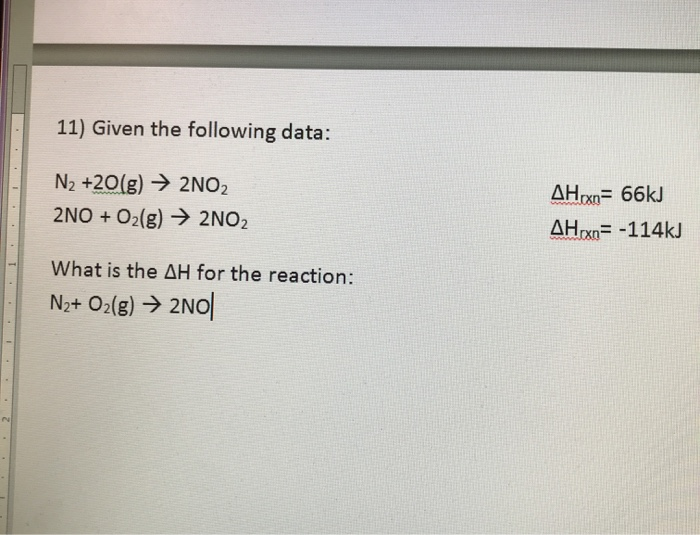11) Given the following data: N2 +20(g) + 2NOZ 2NO + O2(g) → 2NO2 AHxn= 66kJ AHrxn= -114k) What is the AH for the reaction: N2+ O2(g) → 2N0| 11) Given the following data: N2 +2O2(g) → 2NOZ 2NO + O2(g) → 2N02 AHıxn= 66kJ AHrxn= -114k] What is the AH for the reaction: N2+ O2(g) → 2NO 11) Given the following data: N2 +20_18) + 2NO2 2NO + O2(g) → 2NOZ AHxn= 66kJ AHx= -114k] What is the AH...